Cholula Pyramid - A quest for Sumerian measurements.

The largest pyramid in the world is largely unknown. Resembling a man-made mountain and topped by a Catholic church constructed shortly after the Conquest, it has a base four times larger than the famous pyramid of Giza, but only a fraction of its publicity.The Great Pyramid of Cholula

This is a short excerpt from wikipedia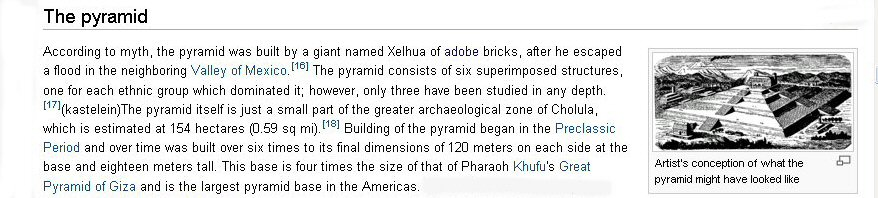Archaeologists studying ancient sites in Mexico have often noticed how some measurements often came out in round numbers in metres. Unknown to them, this is because these sites appear to have been constructed using a measurement system comprising units which in Mesopotamia are called "Sumerian" cubits and in Egypt are called "Egyptian" cubits, the Sumerian cubit of 19.8" is 24/25ths of an Egyptian cubit of 20.625" and it is fairly straightforward to quickly convert metres to Sumerian cubits since 1 metre is virtually 2 Sumerian cubits.

Reading the Wiki article we can quickly spot that the Great Pyramid of Cholula was 120 metres per side, now assuming that it was not built in metres since the metre was not invented until the time of the French Revolution, if we convert to "Sumerian" units we would find that the pyramid measured 240 Sumerian cubits or 360 Sumerian feet per side.

The basic ancient Sumerian measuring units were
shusi = 0.66"
foot = 13.2"
cubit = 19.8"
yard = 33.0"
double yard = 66.0"
rod = 16.5 English feet (15 Sumerian feet)
stade = 100 sumerian cubits (165 English feet)
2 x stade = 200 cubits (330 English feet)
4 x stade =400 cubits (660 English feet or one furlong)
Egyptian royal cubit 20.625"

A plot of 165ft (150 Sumerian feet) was 100 Sumerian cubits and therefore 96 Egyptian cubits, providing very useful combinations for land surveying and division.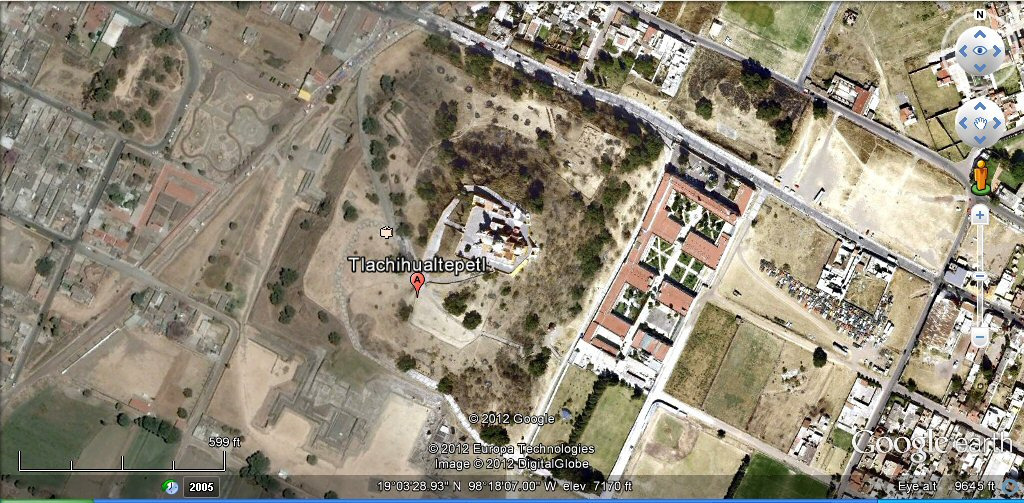Google earth image of the Great Pyramid at Cholula

It is quite often very fifficult to obtain accurate measurements of ancient archaelogical sites, but fortunately for Cholula we have a paper published by Geoffrey G. McCafferty of the Dept of Anthropology, Brown University, USA and I hope he won't mind if I reproduce below his table of dimensions for the Cholula pyramid and plan of the Cholula pyramid.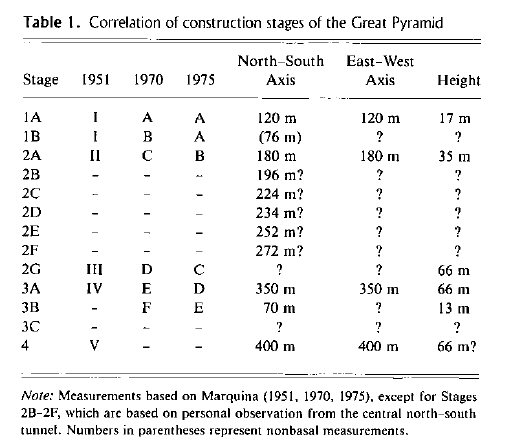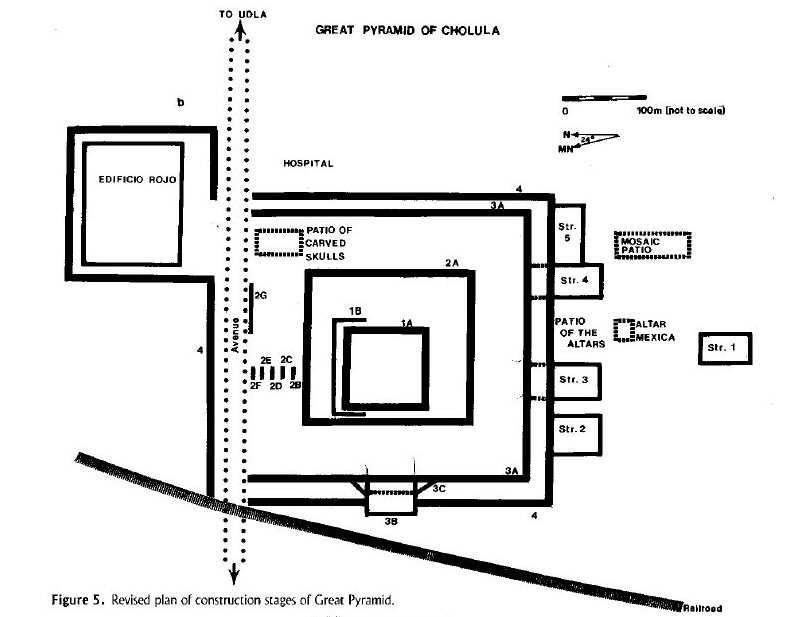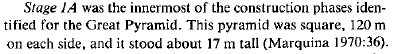We can see that the very first pyramid built had a base side of 120 metres which is 240 Sumerian cubits or 360 Sumerian feet, and a height of 17 metres which is 10 Sumerian double yards, each of 100 Sumerian shusi.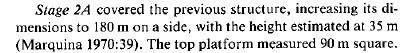When we look at the reconstruction of the pyramid which doubled its size, we can see that the sides have been increased to 180 metres which would be 360 Sumerian cubits, the height 35 metres which would be intended as 20 Sumerian double yards and the platform 90 metres square would be 180 Sumerian cubits square.

Each ancient culture had its own favourite numbering system with favoured numbers of which 360 is a prime example being favoured by Egyptians, Sumerians, and Mexicans as seen above and a target of 360 cubits for the base of a pyramid also occurs in the Akapana pyramid in Tiwanaku, Bolivia which American archaeologist George Squier reported in 1877 as being 188.97 metres in length, which when converted to Egyptian Royal Cubits would be 360 cubits.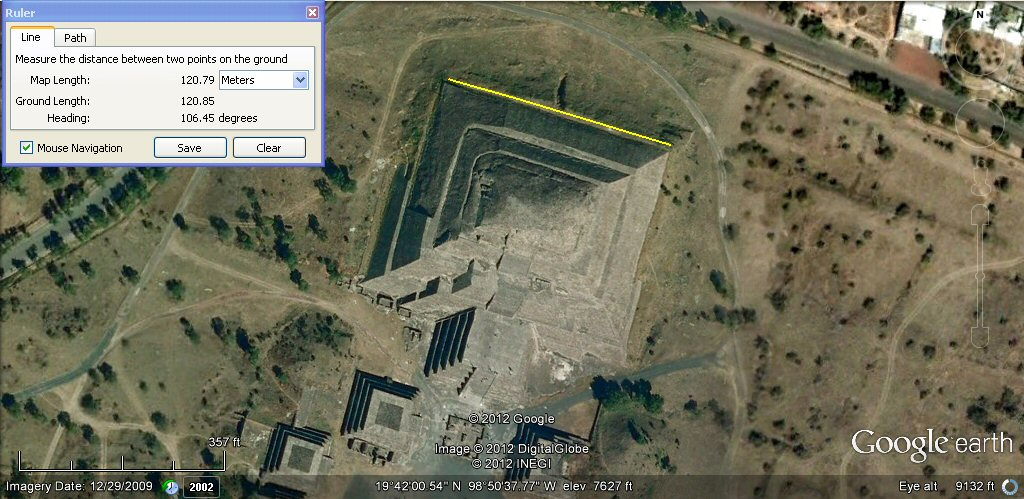Google earth image of the Moon Pyramid at Teotihuacan, above, intended measurement 120.70metres which is 360 Sumerian feet, the same size as phase 1A of the Cholula pyramid.

J.M. Allen, Feb 2012
email webatlantis@hotmail.com